# Lions Weekly: March 2, 2022# A Note From Mr. Sandefur

Lion Family,

As we are blessed to continue to offer Christ-centered education this week, I cannot help but be burdened by the conflicts that are present throughout our world. Please join me in praying for the people of Ukraine that are experiencing terror at this very moment. This moment in world history is yet another reminder of the blessings that we experience through our freedom in this nation. It is my personal desire that we would seek to embrace attitudes of gratitude for all that we have as we pray for those who are less fortunate. While the conflict in Ukraine feels millions of miles away, there are those who walk our campus every day with conflicts present in their own lives. Please join me in praying for the Lord’s provision and unity of His body as we seek to develop servant leaders who will change the world for Christ.

Finally, I would like to encourage you to attend this Saturday’s “Forged" Parent Conference in Woody Auditorium from 9:00 a.m. – 12:00 p.m. As an institution, it is our desire to invest in and develop deeply rooted biblical worldviews in all stakeholders, and this opportunity is aimed at doing so in the arena of parenting in a post-Christian society. We are blessed to have local pastors who will be sharing from scripture and their hearts on topics relevant to parenting within the culture in which we live. I hope that you will be able to join us as we focus on these ever-important topics.

In Christ Alone,

Jeremy Sandefur
President

 table div table+table+table+table div table{width:100%;padding:0}table div table+table+table+table div table img{width:96.23%;padding:0;float:none}table div table+table+table+table div table td{width:100%;padding:0 1.88% 18px}/* styles */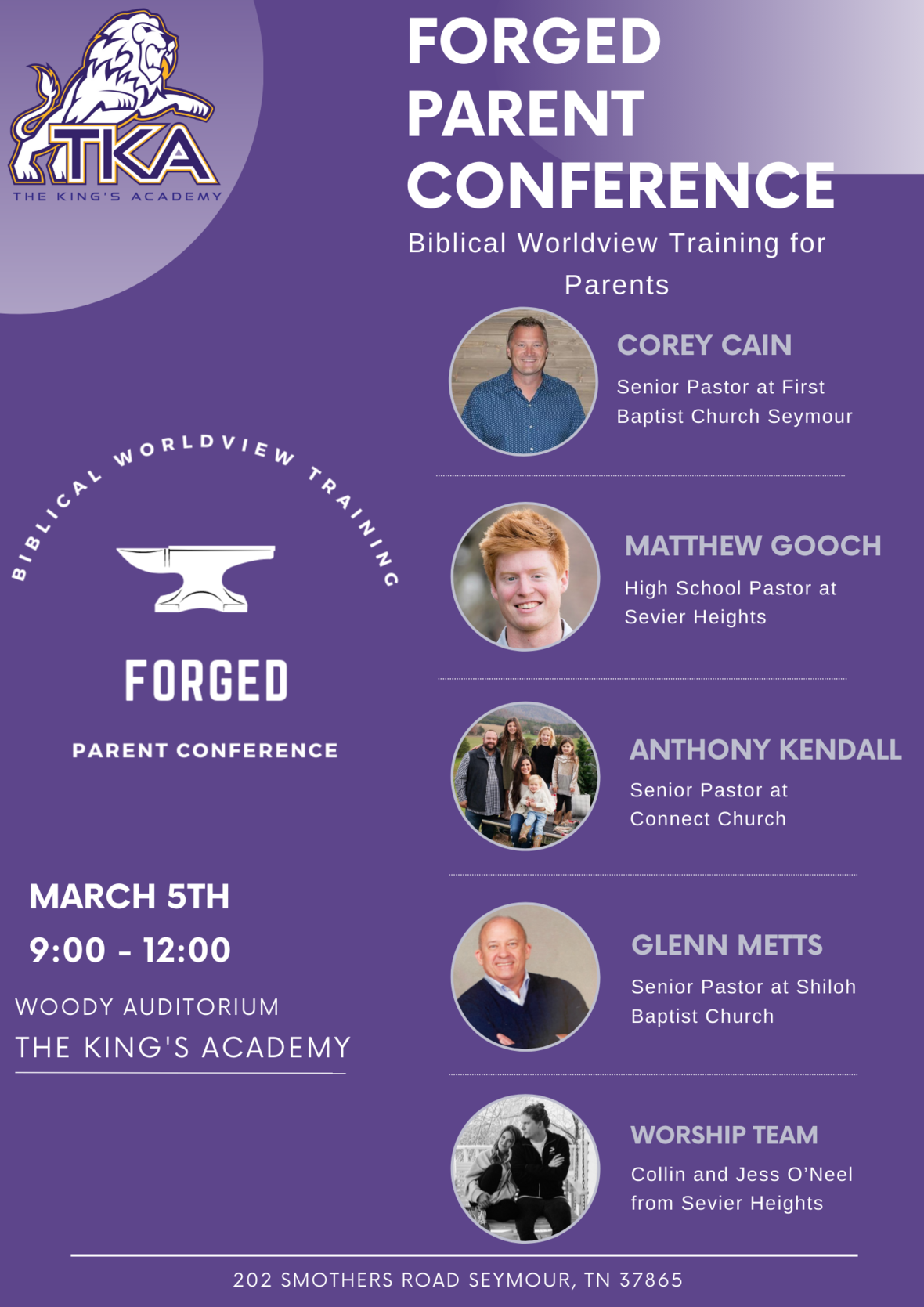# Saturday, March 5

The office of Student Life at The King’s Academy is committed to providing opportunities for our students and their families to develop a Biblical worldview as they face the challenges of our post-Christian society. I would like to personally invite all families to join us this Saturday, March 5th, from 9:00 a.m. - 12:00 p.m. for the “Forged” Parent Conference.

The purpose of “Forged” is to allow iron to sharpen iron as a community with the focus on parenting from a biblical worldview. We are blessed to be led in times of worship and teaching by local pastors as we tackle needed and difficult topics regarding how to lead children through trying times such as our nation currently faces. I fully believe that this three hour investment will be well worth your time as we discuss topics such as discipleship, technology, and the importance of Biblical communication and conflict resolution.

Please prayerfully consider joining us in Woody Auditorium this Saturday morning for “Forged”!

 table div table+table+table+table+table+table+table div table{width:100%;padding:0}table div table+table+table+table+table+table+table div table img{width:96.23%;padding:0;float:none}table div table+table+table+table+table+table+table div table td{width:100%;padding:0 1.88% 18px}/* styles */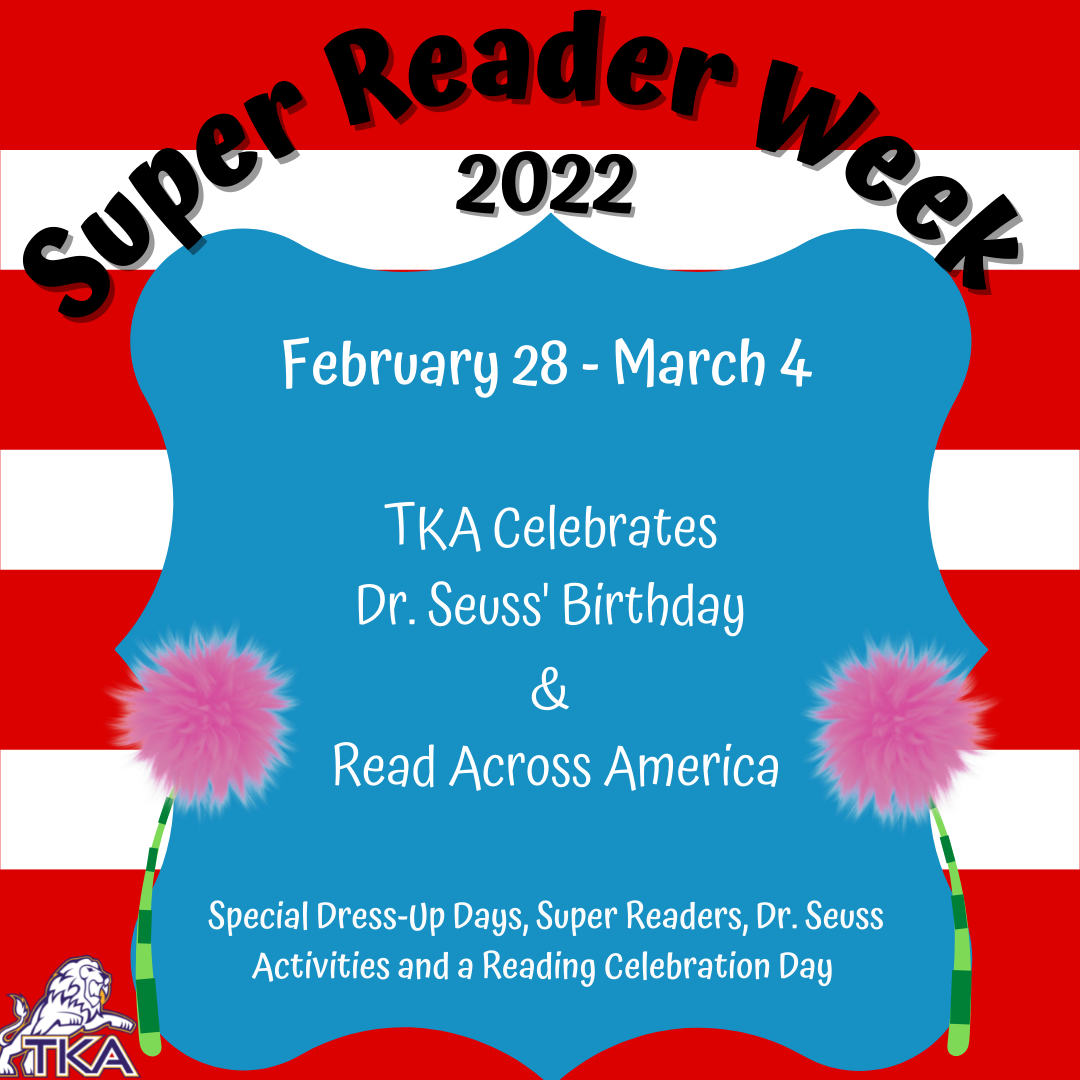We are having a blast this week celebrating the importance of reading and our Little Lion readers! This week coincides with Read Across America Week highlighting Dr. Seuss’ birthday.

My encouragement to all parents is to never take the special 20 minute reading time each night for granted. It is not only a special bonding moment with your child, but it is strengthening your child in so many beneficial ways for a successful academic future.

It is a joy to be a part of a school that takes great pride in developing strong readers. We are beyond blessed to share our love for reading with your child(ren) every day.

 table div table+table+table+table+table+table+table+table+table div table{width:100%;padding:0}table div table+table+table+table+table+table+table+table+table div table img{width:96.23%;padding:0;float:none}table div table+table+table+table+table+table+table+table+table div table td{width:100%;padding:0 1.88% 18px}/* styles */We appreciate her taking time to come read with our Little Lions and sharing the importance of reading in life along with study skills to be a lifelong learner. Maddie also shared her love of Christ with our students and how He has a specific plan and purpose for each one of them.

All elementary students went home with an autographed photo of our Super Reader!

Take a moment and check out some of our Super Readers we have had on campus this week!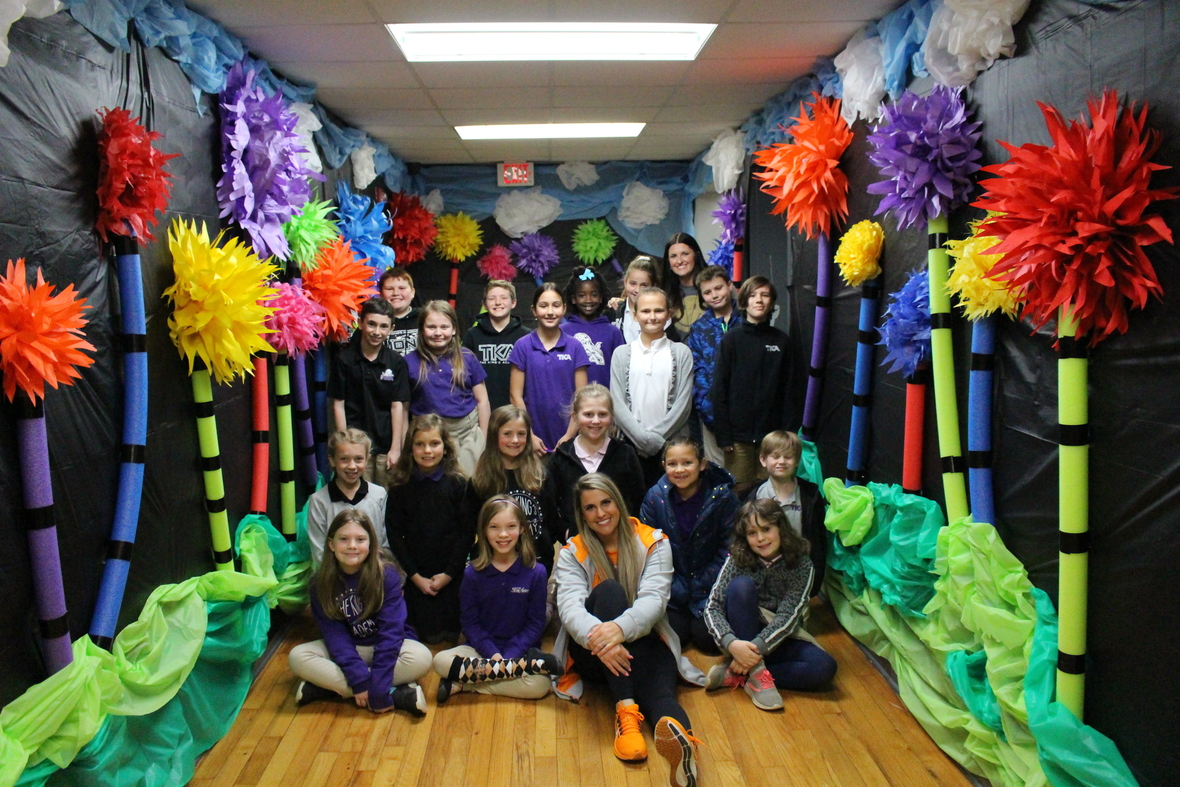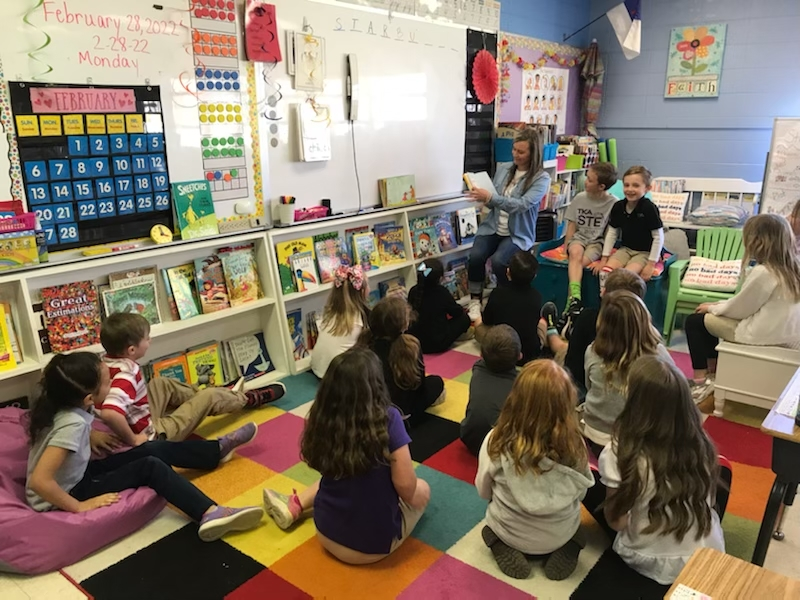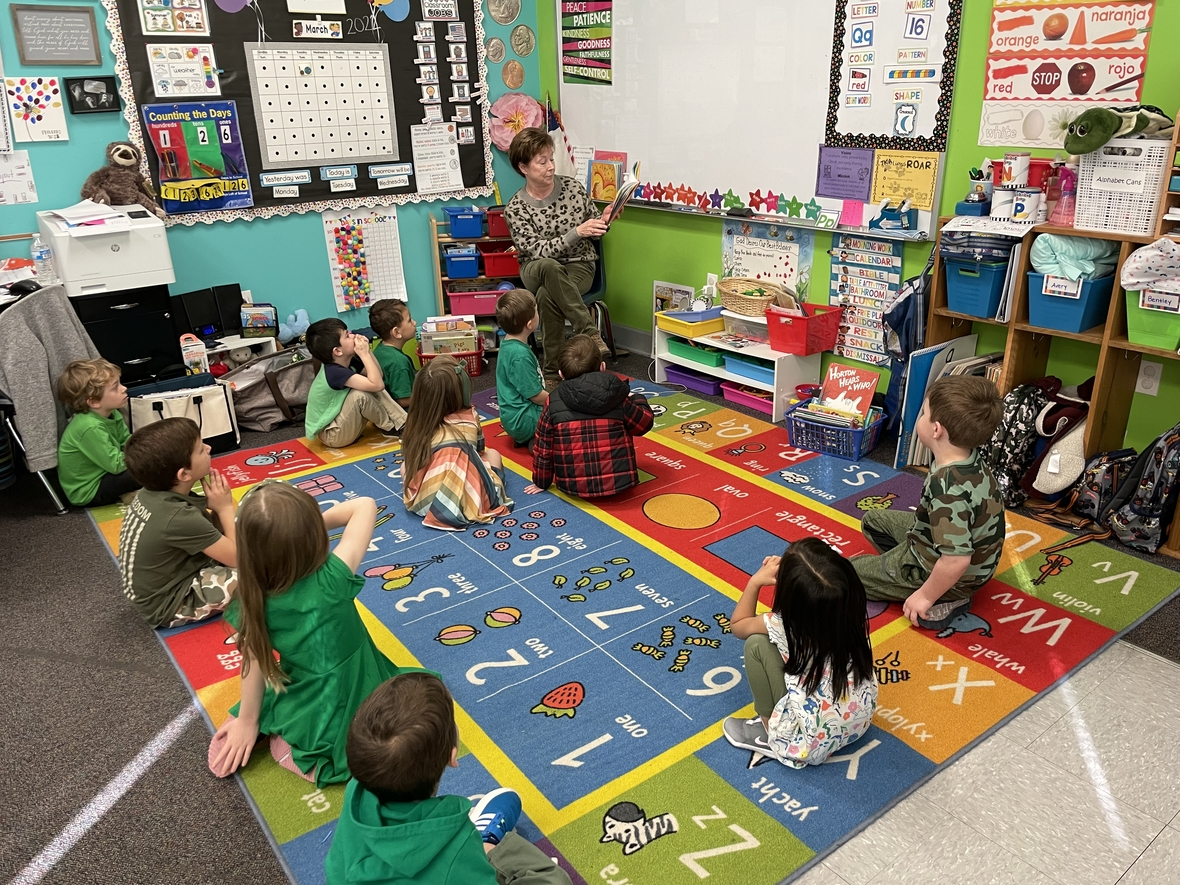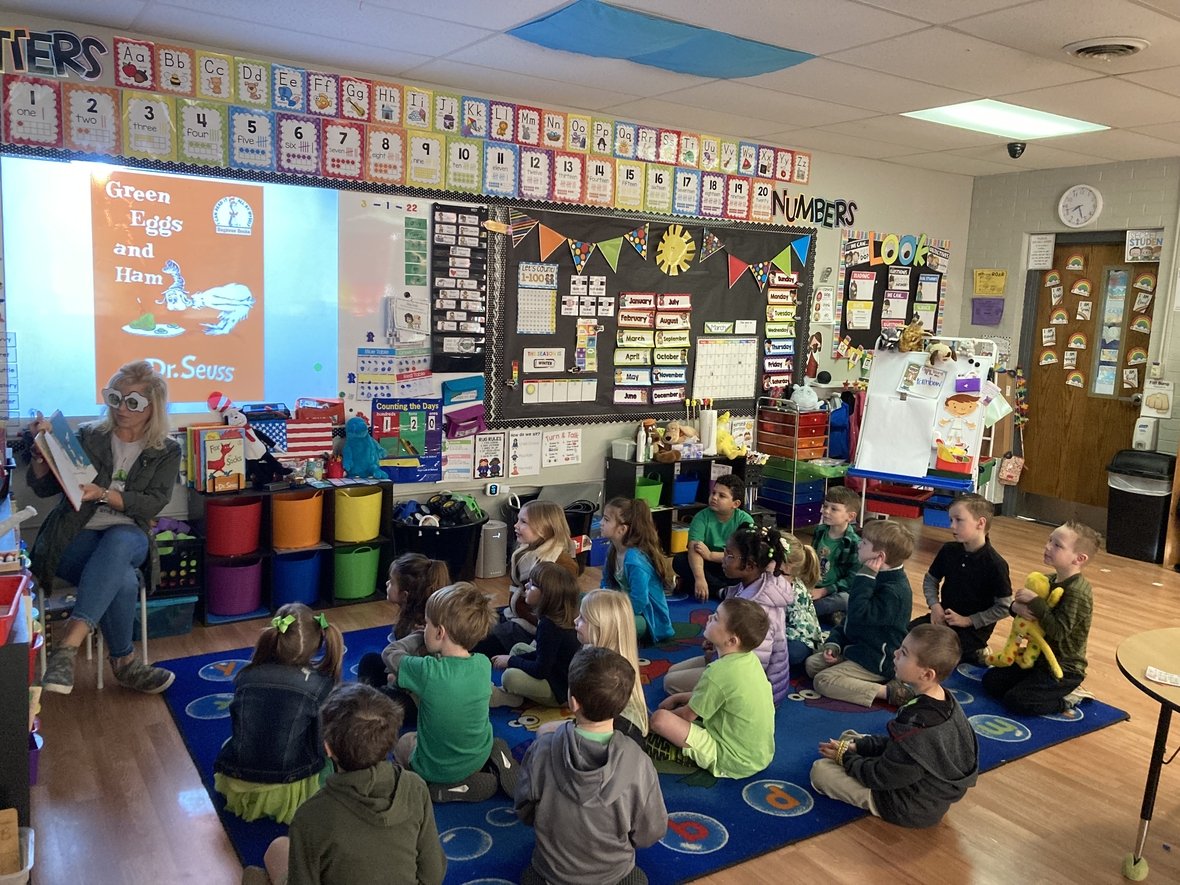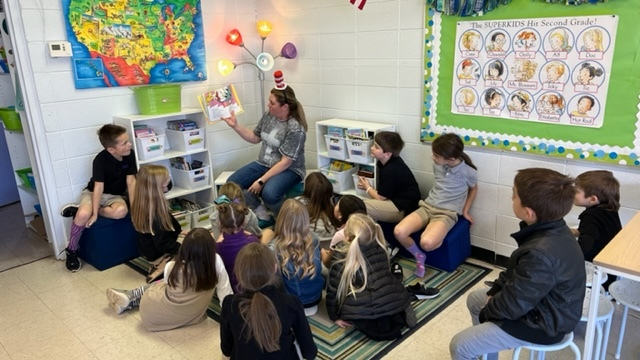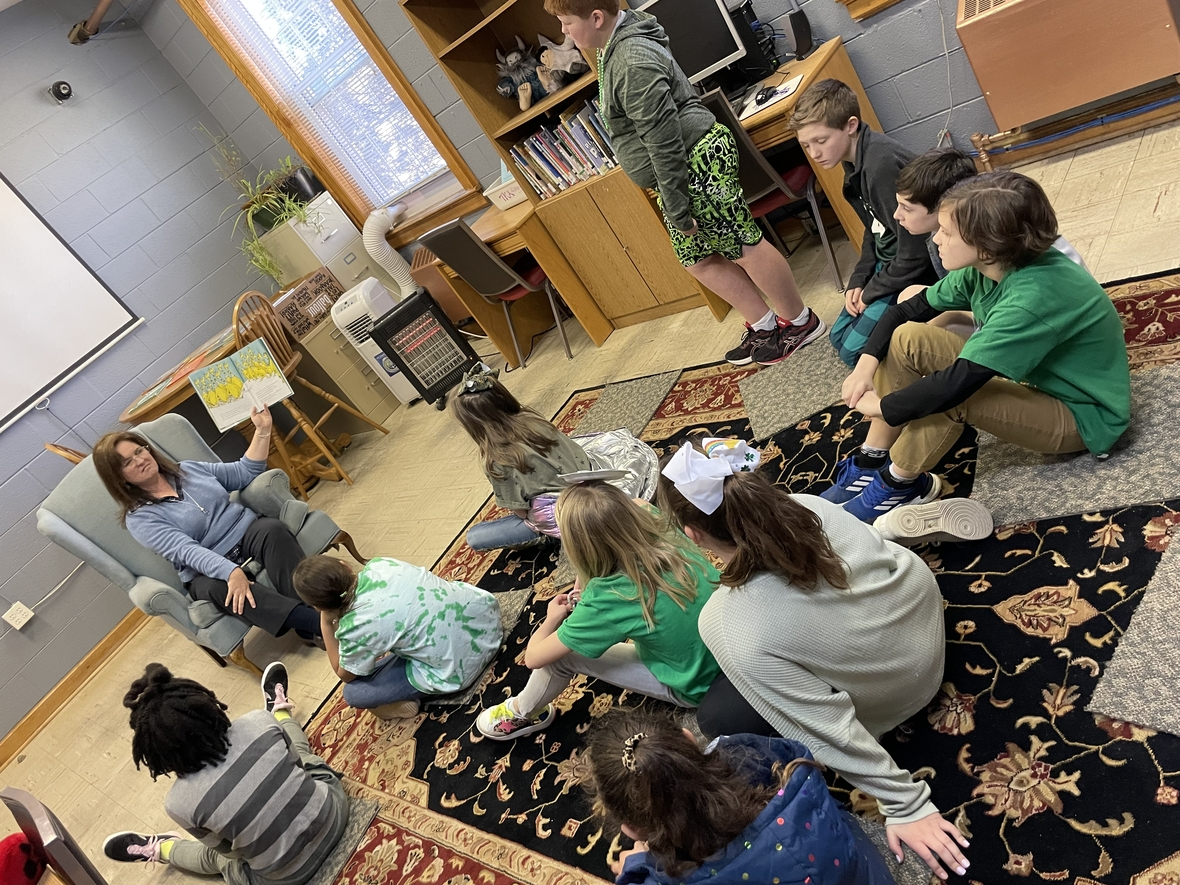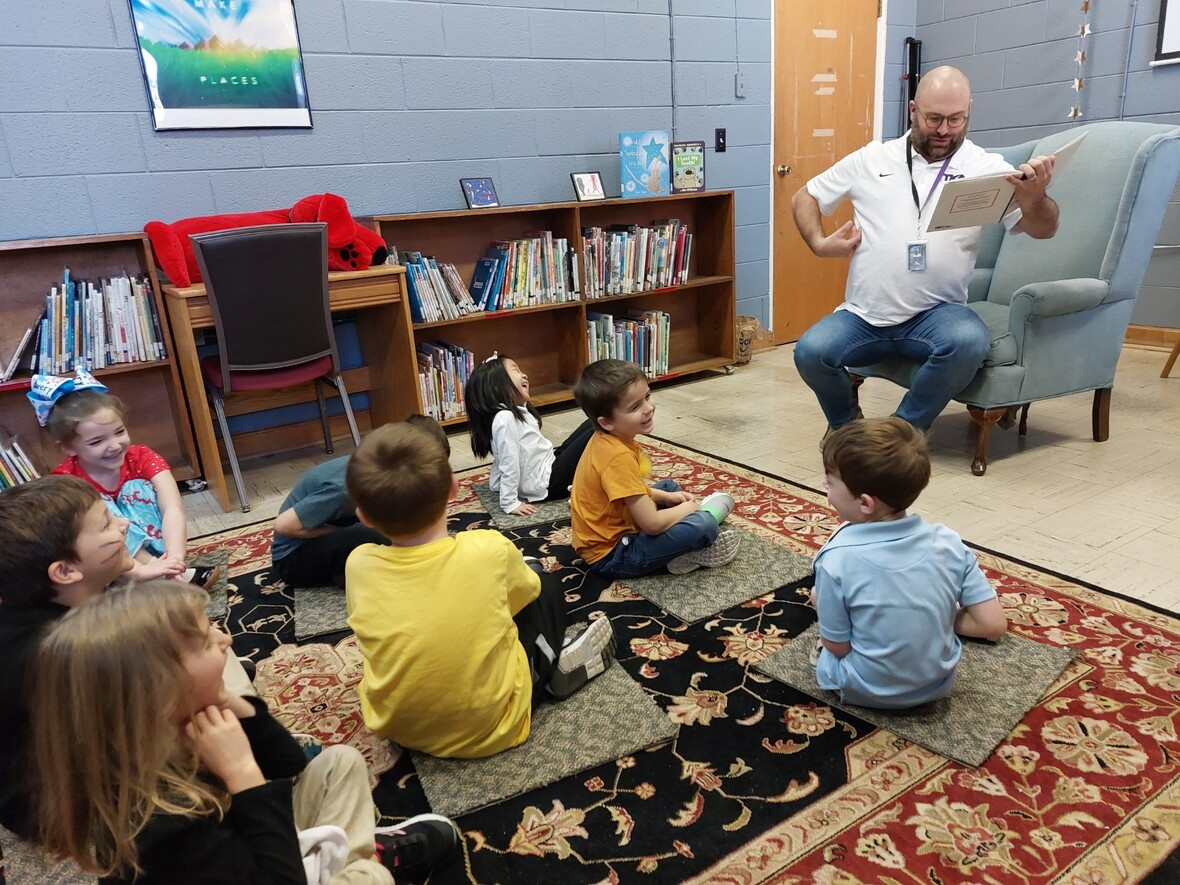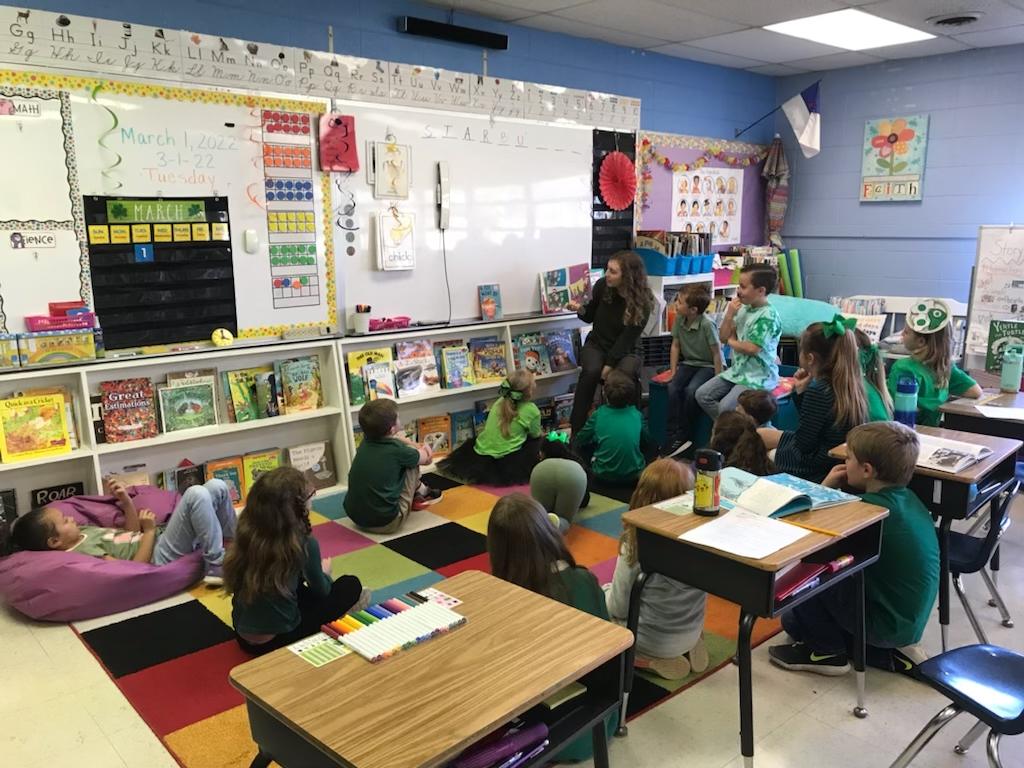table div table+table+table+table+table+table+table+table+table+table+table+table+table+table+table div table{width:100%;padding:0}table div table+table+table+table+table+table+table+table+table+table+table+table+table+table+table div table img{width:96.23%;padding:0;float:none}table div table+table+table+table+table+table+table+table+table+table+table+table+table+table+table div table td{width:100%;padding:0 1.88% 18px}/* styles */# Elementary Super Reader Dress Up Days

## Monday, February 28 - Friday,March 4

Remember, all attire must adhere to dress code.table div table+table+table+table+table+table+table+table+table+table+table+table+table+table+table+table+table+table+table div table{width:100%;padding:0}table div table+table+table+table+table+table+table+table+table+table+table+table+table+table+table+table+table+table+table div table img{width:96.23%;padding:0;float:none}table div table+table+table+table+table+table+table+table+table+table+table+table+table+table+table+table+table+table+table div table td{width:100%;padding:0 1.88% 18px}/* styles */# Spring Book Fair

The spring book fair is open this week! Stop by and shop at the times listed here. If you would like to preview some of the items sold at the book fair, click this link.

 table div table+table+table+table+table+table+table+table+table+table+table+table+table+table+table+table+table+table+table+table+table div table{width:100%;padding:0}table div table+table+table+table+table+table+table+table+table+table+table+table+table+table+table+table+table+table+table+table+table div table img{width:96.23%;padding:0;float:none}table div table+table+table+table+table+table+table+table+table+table+table+table+table+table+table+table+table+table+table+table+table div table td{width:100%;padding:0 1.88% 18px}/* styles */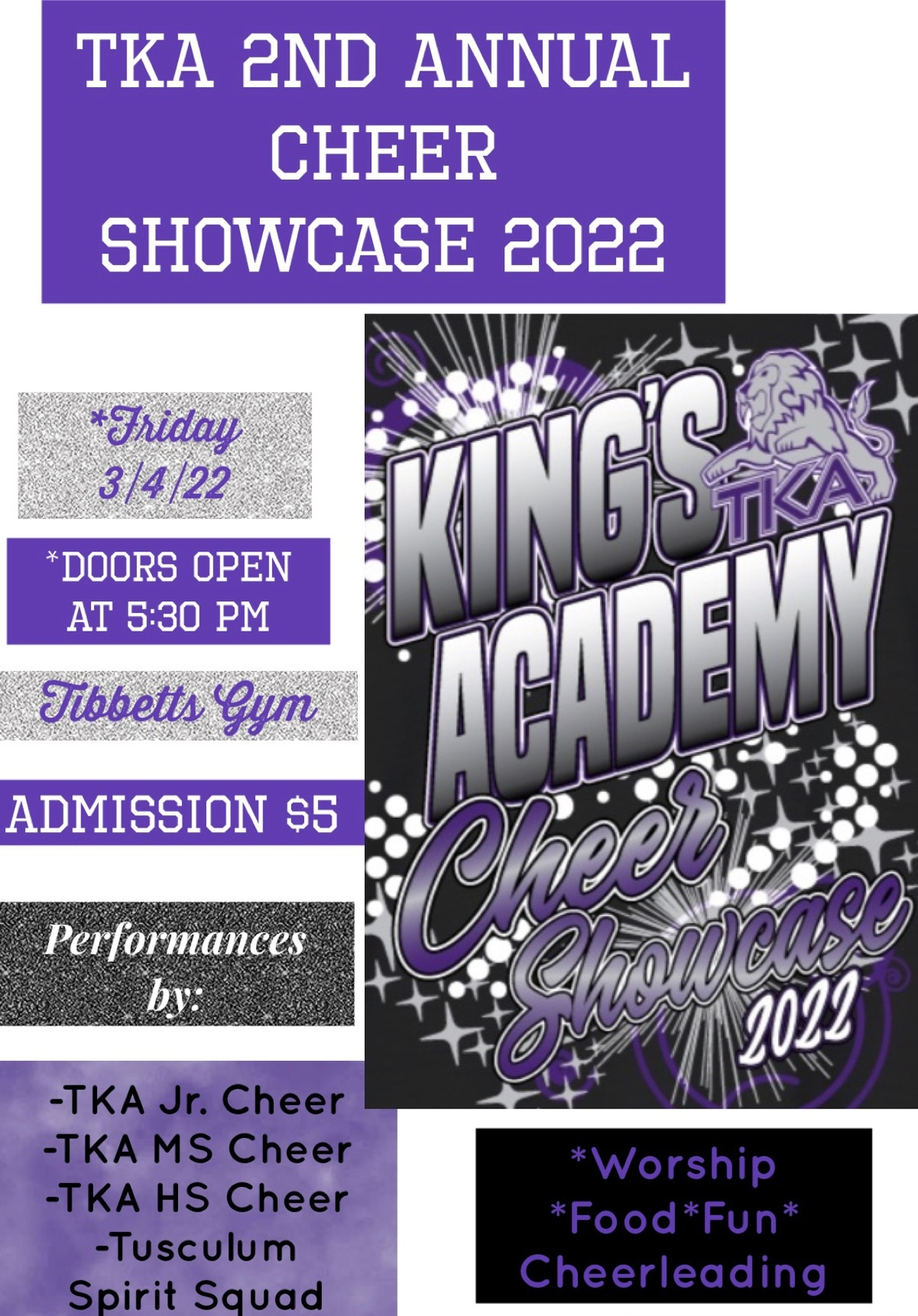# 2022 Cheer Showcase

Mark your calendars for the Cheer showcase on Friday, March 4!

The cheer showcase is a special time to show off all our cheer programs and what they have learned all year. Our K-5 Lions all the way up to high school cheer will perform!

We will also have the Tusculum University Spirit Squad do an exhibition performance and recruitment talk.

We are looking forward to a fun night!

 table div table+table+table+table+table+table+table+table+table+table+table+table+table+table+table+table+table+table+table+table+table+table+table div table{width:100%;padding:0}table div table+table+table+table+table+table+table+table+table+table+table+table+table+table+table+table+table+table+table+table+table+table+table div table img{width:96.23%;padding:0;float:none}table div table+table+table+table+table+table+table+table+table+table+table+table+table+table+table+table+table+table+table+table+table+table+table div table td{width:100%;padding:0 1.88% 18px}/* styles */# Save the Date!

The 9th - 12th grade prom will take place on Saturday, April 9 from 6:30 p.m. - 10:30 p.m. at the Capitol Theatre in Maryville.

More information regarding ticket purchases, outside guest permission forms, and dress check dates will be coming soon!

 table div table+table+table+table+table+table+table+table+table+table+table+table+table+table+table+table+table+table+table+table+table+table+table+table+table div table{width:100%;padding:0}table div table+table+table+table+table+table+table+table+table+table+table+table+table+table+table+table+table+table+table+table+table+table+table+table+table div table img{width:96.23%;padding:0;float:none}table div table+table+table+table+table+table+table+table+table+table+table+table+table+table+table+table+table+table+table+table+table+table+table+table+table div table td{width:100%;padding:0 1.88% 18px}/* styles */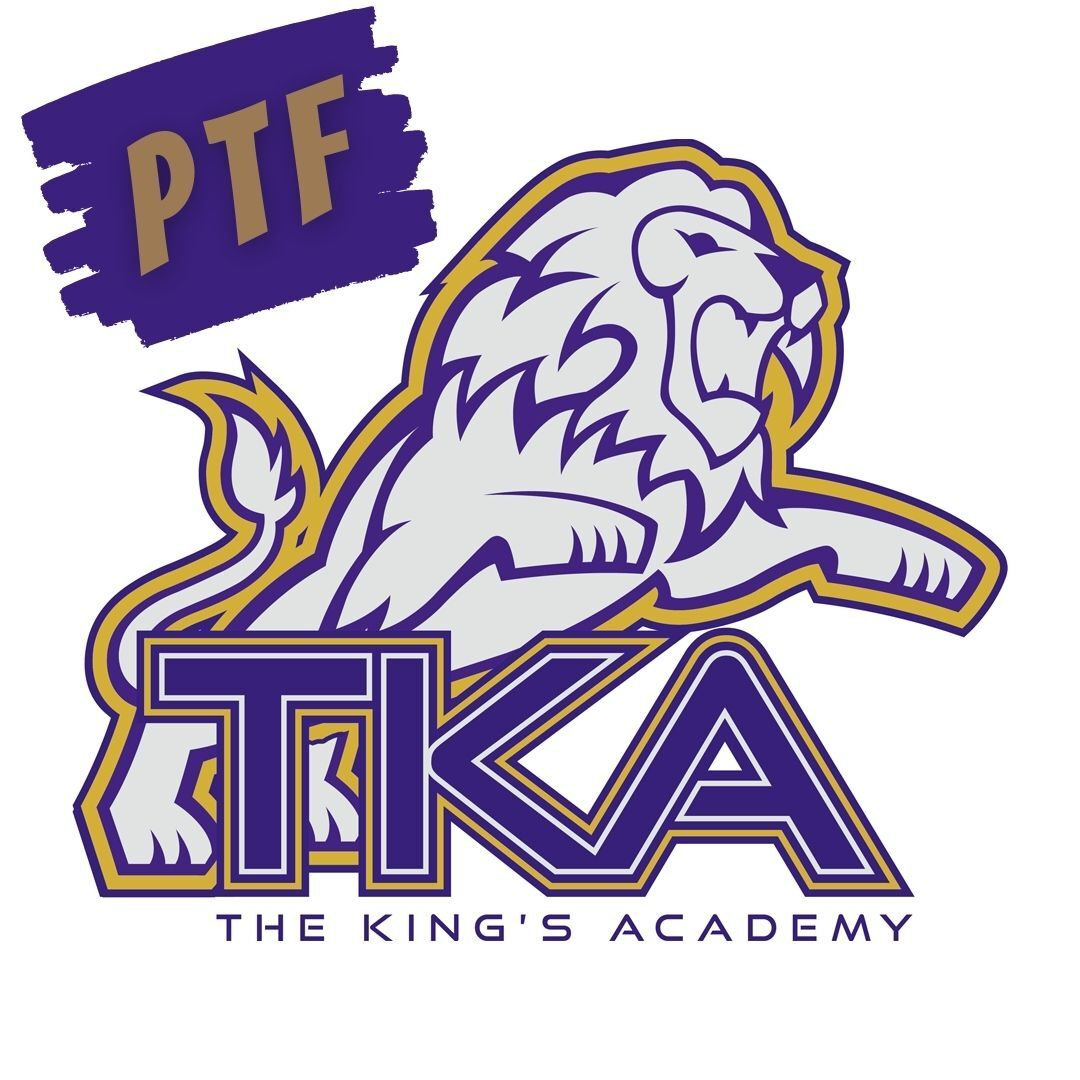# Upcoming PTF Dates

Below are the upcoming PTF meetings for the remainder of the year from 3:15 - 4:15 in Brandes:

Thursday, March 31
Thursday, April 28
* Thursday, May 19

PTF will also host a Mother/Son kickball event on Saturday, April 30, at 12:00 p.m. More information is to come in the days ahead!

 table div table+table+table+table+table+table+table+table+table+table+table+table+table+table+table+table+table+table+table+table+table+table+table+table+table+table+table div table{width:100%;padding:0}table div table+table+table+table+table+table+table+table+table+table+table+table+table+table+table+table+table+table+table+table+table+table+table+table+table+table+table div table img{width:96.23%;padding:0;float:none}table div table+table+table+table+table+table+table+table+table+table+table+table+table+table+table+table+table+table+table+table+table+table+table+table+table+table+table div table td{width:100%;padding:0 1.88% 18px}/* styles */# Elementary Summer Tutoring

Dear TKA Families,

My name is Ms. Addison, and I teach third grade here at TKA. I have been a part of the TKA family for three years now, including a portion of my masters degree internship program. I am excited to announce I will be offering summer tutoring for elementary grades in reading, math, and ELA. I will be offering tutoring to better solidify skills from the grade the student will be leaving, or working on skills to prepare for the upcoming school year. The cost will be \$35/hour which can be made payable through the school. I will be working out of my classroom in the Ogle Elementary building.

Please select the dates and times from the Google form below that you are requesting. After the deadline of April 8th, 2022, I will organize the requests, finalize a schedule and reach out to each family in regards to your request. Please know space is limited and not all requests will be able to be fulfilled.

 /* styles */ Thank you in advance for the opportunity to be a part of your student's education. I look forward to a fun and successful summer. -Ms. Addison 3rd Grade Teacher
 table div table+table+table+table+table+table+table+table+table+table+table+table+table+table+table+table+table+table+table+table+table+table+table+table+table+table+table+table+table+table+table div table{width:100%;padding:0}table div table+table+table+table+table+table+table+table+table+table+table+table+table+table+table+table+table+table+table+table+table+table+table+table+table+table+table+table+table+table+table div table img{width:96.23%;padding:0;float:none}table div table+table+table+table+table+table+table+table+table+table+table+table+table+table+table+table+table+table+table+table+table+table+table+table+table+table+table+table+table+table+table div table td{width:100%;padding:0 1.88% 18px}/* styles */# Upcoming Sports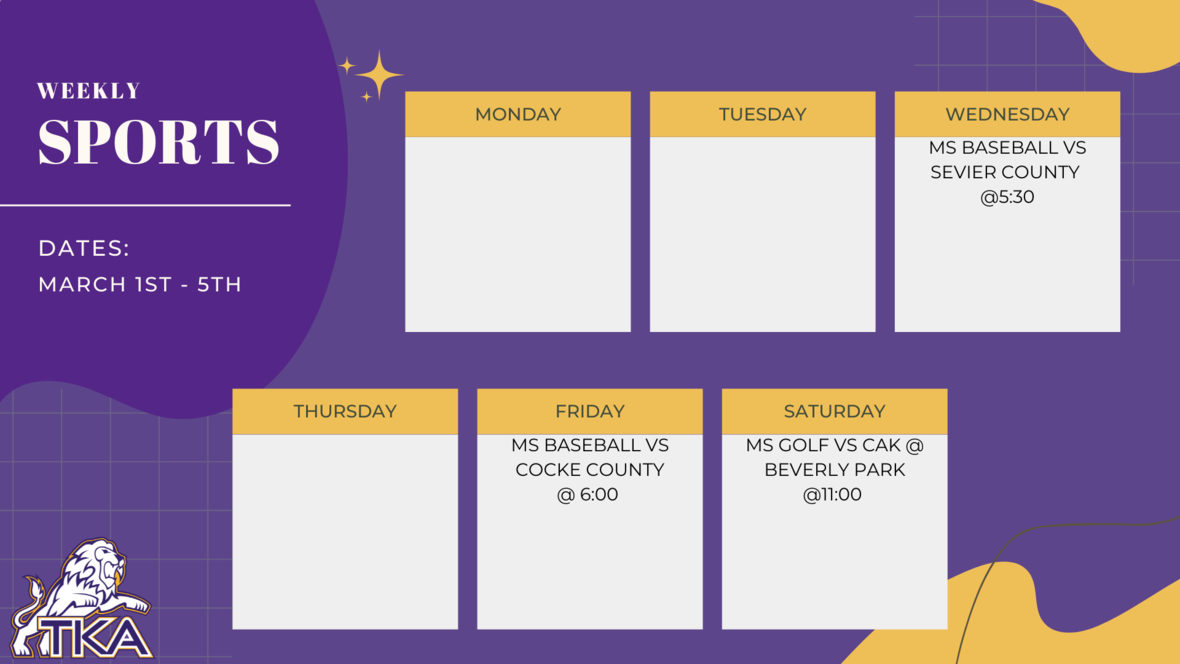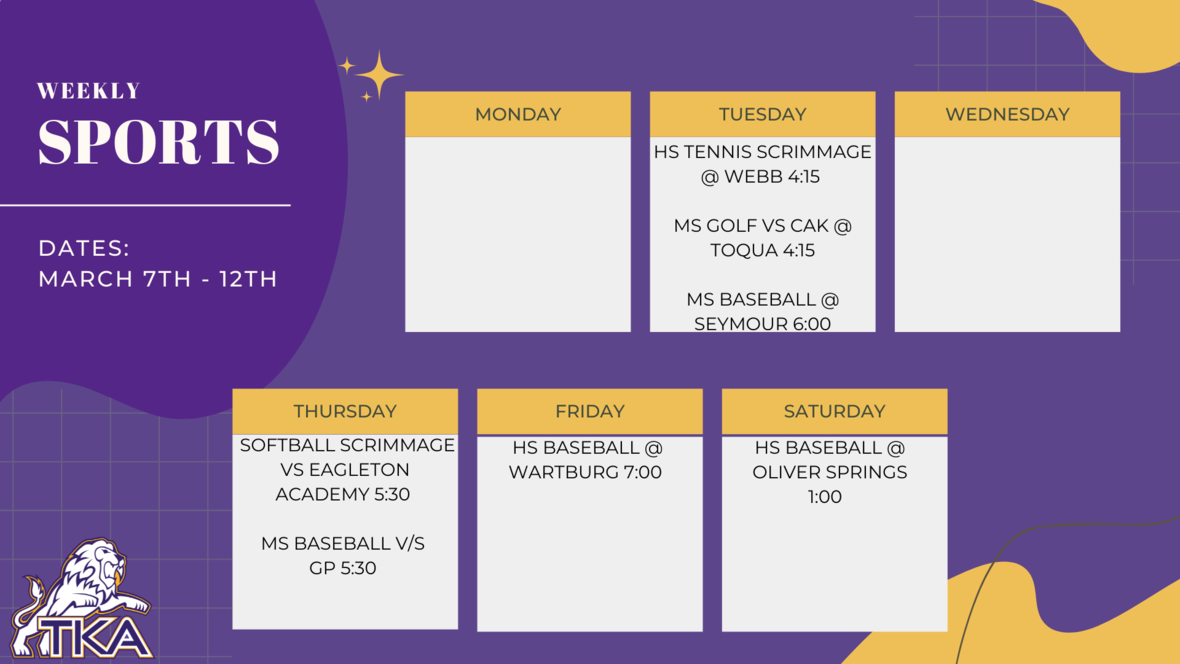table div table+table+table+table+table+table+table+table+table+table+table+table+table+table+table+table+table+table+table+table+table+table+table+table+table+table+table+table+table+table+table+table+table+table+table div table{width:100%;padding:0}table div table+table+table+table+table+table+table+table+table+table+table+table+table+table+table+table+table+table+table+table+table+table+table+table+table+table+table+table+table+table+table+table+table+table+table div table img{width:96.23%;padding:0;float:none}table div table+table+table+table+table+table+table+table+table+table+table+table+table+table+table+table+table+table+table+table+table+table+table+table+table+table+table+table+table+table+table+table+table+table+table div table td{width:100%;padding:0 1.88% 18px}/* styles */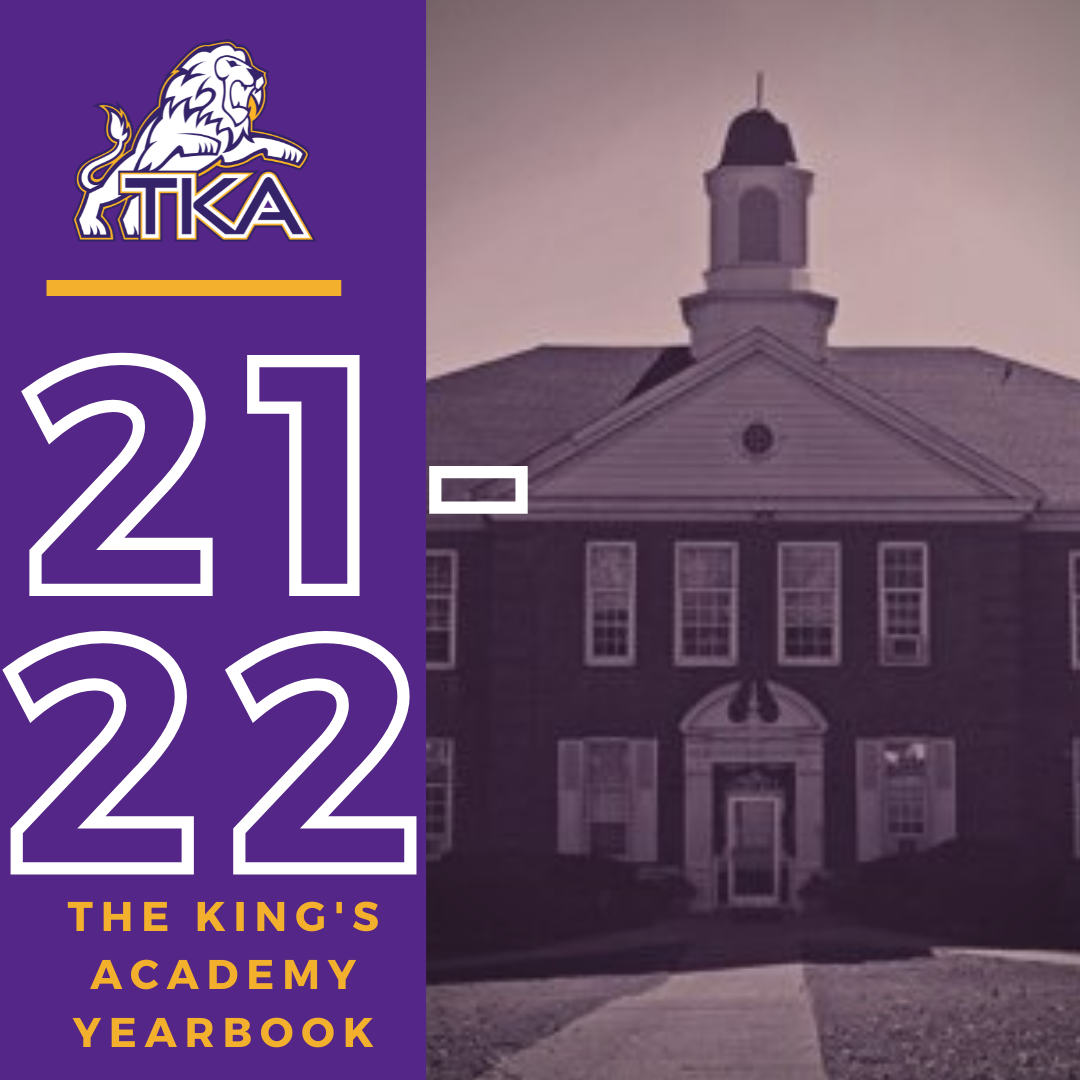# 2021-2022 Yearbook

The price for the 2021-2022 TKA yearbook is \$55. All grades, K3-12 will have the same yearbook. The slight price increase is because we are only going to have one larger all-school book.

All yearbook orders can be placed online--here is the link.Do you have a class of 2022 senior in your household?

Don't miss out on the opportunity to purchase a Senior Tribute for the 2021-2022 yearbook!

Senior Tributes are available for purchase at the bottom of the link listed here.For any questions regarding yearbooks, email Mr. Josh Smith at jsmith@thekingsacademy.net.

 table div table+table+table+table+table+table+table+table+table+table+table+table+table+table+table+table+table+table+table+table+table+table+table+table+table+table+table+table+table+table+table+table+table+table+table+table+table+table+table div table{width:100%;padding:0}table div table+table+table+table+table+table+table+table+table+table+table+table+table+table+table+table+table+table+table+table+table+table+table+table+table+table+table+table+table+table+table+table+table+table+table+table+table+table+table div table img{width:96.23%;padding:0;float:none}table div table+table+table+table+table+table+table+table+table+table+table+table+table+table+table+table+table+table+table+table+table+table+table+table+table+table+table+table+table+table+table+table+table+table+table+table+table+table+table div table td{width:100%;padding:0 1.88% 18px}/* styles *//* styles */
 table div table+table+table+table+table+table+table+table+table+table+table+table+table+table+table+table+table+table+table+table+table+table+table+table+table+table+table+table+table+table+table+table+table+table+table+table+table+table+table+table+table+table div table{width:100%;padding:0}table div table+table+table+table+table+table+table+table+table+table+table+table+table+table+table+table+table+table+table+table+table+table+table+table+table+table+table+table+table+table+table+table+table+table+table+table+table+table+table+table+table+table div table img{width:96.23%;padding:0;float:none}table div table+table+table+table+table+table+table+table+table+table+table+table+table+table+table+table+table+table+table+table+table+table+table+table+table+table+table+table+table+table+table+table+table+table+table+table+table+table+table+table+table+table div table td{width:100%;padding:0 1.88% 18px}/* styles */# Covid-19 Procedures

While we are excited to offer in-person education, we must maintain a safe learning environment for all students, faculty/staff, and additional stakeholders.

To do so, we will be working closely with the Sevier County Health Department regarding Covid-19 protocols.

 table div table+table+table+table+table+table+table+table+table+table+table+table+table+table+table+table+table+table+table+table+table+table+table+table+table+table+table+table+table+table+table+table+table+table+table+table+table+table+table+table+table+table+table+table div table{width:100%;padding:0}table div table+table+table+table+table+table+table+table+table+table+table+table+table+table+table+table+table+table+table+table+table+table+table+table+table+table+table+table+table+table+table+table+table+table+table+table+table+table+table+table+table+table+table+table div table img{width:96.23%;padding:0;float:none}table div table+table+table+table+table+table+table+table+table+table+table+table+table+table+table+table+table+table+table+table+table+table+table+table+table+table+table+table+table+table+table+table+table+table+table+table+table+table+table+table+table+table+table+table div table td{width:100%;padding:0 1.88% 18px}/* styles */table div table+table+table+table+table+table+table+table+table+table+table+table+table+table+table+table+table+table+table+table+table+table+table+table+table+table+table+table+table+table+table+table+table+table+table+table+table+table+table+table+table+table+table+table+table+table div table{width:100%;padding:0}table div table+table+table+table+table+table+table+table+table+table+table+table+table+table+table+table+table+table+table+table+table+table+table+table+table+table+table+table+table+table+table+table+table+table+table+table+table+table+table+table+table+table+table+table+table+table div table img{width:96.23%;padding:0;float:none}table div table+table+table+table+table+table+table+table+table+table+table+table+table+table+table+table+table+table+table+table+table+table+table+table+table+table+table+table+table+table+table+table+table+table+table+table+table+table+table+table+table+table+table+table+table+table div table td{width:100%;padding:0 1.88% 18px}/* styles */